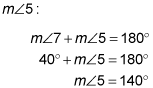##### Geometry: 1,001 Practice Problems For Dummies (+ Free Online Practice)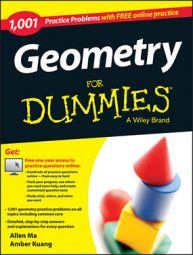When two parallel lines are intersected by a third line (called a transversal), congruent pairs of angles are formed, including alternate interior angles and alternate exterior angles.

The following practice questions ask you to use this information to find a missing angle, and then to apply some algebra to calculate a missing variable.

## Practice questions

Use the diagram and the given information to solve the problem.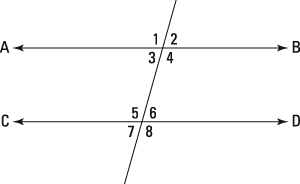1. Parallel lines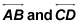are cut by a transversal. If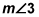is represented by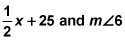is represented by 4x – 45, find the value of x.

2. Parallel linesare cut by a transversal. If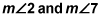are represented by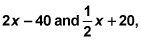respectively, find the degree measure of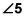## Answers and explanations

1. 20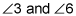are alternate interior angles. Alternate interior angles are congruent, so set their measures equal to each other and solve for x: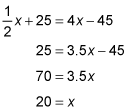2. 140 degrees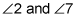are alternate exterior angles. Alternate exterior angles are congruent, so set their measures equal to each other and solve for x: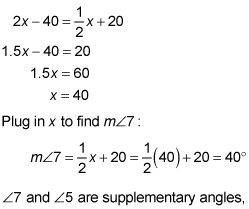which means they add up to 180 degrees. Use this info to solve for